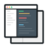# Implement Back-Propagation Algorithm for Classification Problems

##### In this machine learning project, we will implement Back-propagation Algorithm from scratch for classification problems.#### Videos

Each project comes with 2-5 hours of micro-videos explaining the solution.#### Code & Dataset## What will you learn

What are Artificial Neural Networks
Backpropagation and Forwardpropagation
Structure of a Neural Network (Neuron)
Input feature Weight Vector, Sum Function, Activation Function, and Bias in the network
Defining an activation function and understanding different types of activation function
Back Propagation NN is the multilayered feedforward NN
Steps in backpropagation algorithm, defining weights forward feeding to get output, and error backpropagation
Defining a function for Initializing the network
Calculating the neuron activation for an input
Defining the Transfer function for neuron activation
Defining function for forwarding propagate input to a network output
Testing the forward propagation
Calculating the derivative of a neuron output
Backpropagating the error and storing it in neurons
Updating the network weights with calculated error
Training the network with some iterations
Importing the final dataset for testing created algorithm
Preprocessing the dataset and scaling it for better results
Feeding the dataset into Artificial Neural Networks and calculating the results
Applying cross-validation to prevent overfitting
Making the final predictions and calculating the accuracy score

## Project Description

From the perspective of risk management, the result of predictive accuracy of the estimated probability of default will be more valuable than the binary result of classification - credible or not credible clients. Because the real probability of default is unknown, so in this machine learning project we present the novel Sorting Smoothing Method to estimate the real probability of default.

With the real probability of default as the response variable (Y), and the predictive probability of default as the independent variable (X), the simple linear regression result (Y = A + BX) shows that the forecasting model produced by artificial neural network has the highest coefficient of determination; its regression intercept (A) is close to zero, and regression coefficient (B) to one. Therefore, among the six data mining techniques, artificial neural network is the only one that can accurately estimate the real probability of default.

## Similar Projects

#### Bosch Production Line Performance Data Science Project

In this data science project, we will predict internal failures of Bosch using thousands of measurements and tests made for each component along the assembly line.

#### Machine Learning project for Retail Price Optimization

In this machine learning pricing project, we implement a retail price optimization algorithm using regression trees. This is one of the first steps to building a dynamic pricing model.

#### Human Activity Recognition Using Smartphones Data Set

In this deep learning project, you will build a classification system where to precisely identify human fitness activities.

4-Dec-2016
04h 44m